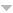# Library

Author TitleMacMillan W.D. Theoretical Mechanics Statics and the Dynamics of a Particle
Heider L.J., Simpson J.E. Theoretical Analysis
Heinkel B. Théorèmes Limites Dans les Espaces a Base Inconditionnelle.
Ramis J.-P. Théorèmes d'Indices Gevrey pour les Equations Différentielles Ordinaires.
Jouanolou J.P. Theoremas de Bertini et applications
Titchmarsh E.C. The Zeta-function of Riemann
Warshauer M.L. The witt group of degree k maps and asymmetric inner product spaces
Lanczos C. The variational principles of mechanics.
Williamson S.G. The Tree oOf Paths Of A Graph
Flicker Y.Z. The trace formula and base change for GL(3).
NULL The Thorndike Barnhart handy pocket Dictionary.
Schilling, O.F.G. The theory of valuations
Mackey G.W. The Theory of Unitary Group Representations.
Cox D.R., Miller H.D. The Theory of Stochastic Processes
Jacobson N. The Theory of Rings
Sternberg W.J. and Smith T.L. The theory of potentials and spherical harmonics
Carmichael R.D. The theory of numbers and Diophantine analysis. Both Books bound as one.
Ahlfors L.W. The Theory of Meromorphic Curves
Gantmacher F.R. The theory of matrices. Vol. 2.
Yano K. The theory of lie derivatives and its applications
Hartman S., Mikusinski J. The theory of Lebesgue measure and integration.
Dixon P.B. The Theory of Joint Maximization
Littlewood D.E. The theory of group characters and matrix representations of groups.
Graves L.M. The theory of functions of real variables.
Sveshnikov A.G. and Tikhonov A.N. The Theory of Functions of Complex Variabk\le
Hobson E.W. The theory of functions of a real variable and the theory of Fourier series. Vol. II.
Hobson E.W. The theory of functions of a real variable and the theory of Fourier series. Vol. I.
Redei L. The Theory of Finitely Generated Commutative Semigroups.
Burnside William Snow, Panton Authur William The theory of equations: with an introduction to the thoery of binary algebraic forms. V.1-2
Burnside William Snow, Panton Authur William The theory of equations: with an introduction to the thoery of binary algebraic forms. V.1-2
Burnside William Snow, Panton Authur William The theory of equations: with an introduction to the thoery of binary algebraic forms. V.1-2
Burnside William Snow, Panton Authur William The theory of equations: with an introduction to the thoery of binary algebraic forms. V.1-2
Muir Th. The theory of determinants in the historical order of development. Vol III. The period 1861-1880. Vol. IV. The period 1880-1900.
Muir Th. The theory of determinants in the historical order of development. Vol I. General and special determinants up to 1841. Vol. II. The period 1841-1860.
Collingwood E.F., Lohwater A.J. The theory of cluster sets.
Jackson D. The theory of approximation
Hodge W.V.D. The theory and applications of harmonic integrals.
Dienes P. The Taylor series. An Introduction to the Theory of Functions of a Complex Variable.
Dubinsky Ed. The Structure of Nuclear Frechet Spaces.
Mayer D.H. The Ruelle-Araki transfer operator in classical statistical Mechanics.